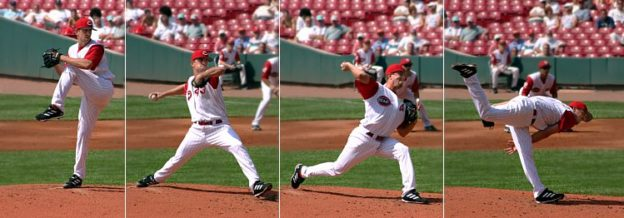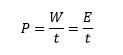Are you more of a visual learner? Check out our online video lectures and start your physics course now for free!Image: ”
Baseball pitching motion 2004.jpg. More details: Baseball pitching motion 2004.” License: CC BY-SA 3.0

## Work-Energy Theorem

W ⇒ work (J or N m)

Work is the energy transfer from one object to another so that the 2nd object can be moved or deformed. As with force, there are several types of work, which are described in the table below.

 Work Equation Description Lifting Work WH WH = FG h Lifting an object at constant speed FG = force of gravity h = height that the object is lifted to Acceleration Work WB WB = FB s FB = m a Change in the velocity of an object, which makes it move faster or slower FB = acceleration s = displacement Pressure-Volume Work wV wV = – ∫ p dV Volume of a fluid is compressed (V2 < V1) or expanded (V1 < V2)

## Power

P ⇒ power (W or J/s)

Power describes the amount of energy consumed per unit of time; i.e., it is dependent on time. The performed work and the incoming energy are inversely proportional to time; i.e., with energy remaining constant, power decreases with time.Name Symbol Units Unit abbreviation Force F Newtons = kg m/s2 N Energy K, U, E Joules = kg m2/s2 J Work W Joules = kg m2/s2 J Power P Watts = J/s = kg m2/s3 W

## Conservative Forces

• Gravity only converts between K and U.

• Total energy remains constant.
• The net work done by gravity only depends on initial and final location.

Work performed by gravity is the same for any path between the same 2 points.

1. WGravity = 0 ↑ ↓

2. WGravity = 0 →
3. WGravity = 0 ↓ ↑  ↑

Work performed by gravity depends on initial and final locations regardless of path. Energy is conserved.

## Nonconservative Forces

Nonconservative forces are forces that do not store energy. They are also known as dissipative forces. For example, when someone pushes a book across a table, the work put into it must be enough to cause the book to move across the table. It must also be enough to combat the friction that occurs in the opposite direction. This nonconservative force does not store energy. The same concept also applies to air resistance when a plane flies through the sky. These friction-type forces remove energy from the system and are no longer available to the system for kinetic energy.

 Conservative forces Nonconservative forces The work done by gravity depends on initial and final locations regardless of path. The work done by friction is path-dependent. Energy is conserved. Energy is not conserved (lost to heat).

### Dependence on path

In a nonconservative force, the work performed is path-dependent. Work is related to the force exerted on the object and the distance the object travels. In other words, Work = Force x Distance. Because friction is a nonconservative force, the amount of work due to friction depends on the distance traveled by the object that has a force exerted on it.

For example, if 2 boxes need to be moved from point A to point B along the same path, the force needed will be the same for each box. However, if Box 1 is moved in a straight line, and Box 2 is moved up and down an incline to reach the same destination, the force of friction for each box will be different.

Because Box 2 took a longer path, the work required to move it will be higher than the work needed to move Box 1. Work is related to the motion of the object plus the work related to friction. Friction always acts in 180 degrees opposite of motion. It acts against the direction of motion and takes away kinetic energy.

In equation form:

Wfriction = F d cos (180) = –Ff d

Mechanical advantage is a measure of the force amplification by using a tool, mechanical device, or machine system. A lever (seesaw) and a pulley system are examples of mechanical advantage. The device preserves the input power and trades off forces against the motion to create the desired amplification.

### Levers

A lever gives the ability to trade off distance for a force for given energy input. In this system, energy is conserved. The force that is applied is used to overcome gravitational energy by pushing (using the force) down on the lever. The lever (seesaw) pivots on a fulcrum. If the fulcrum is placed in the middle of the lever, the force exerted on one side will be equally applied to the other side when lifting the object. In this case, the distance between the fulcrum and the force and the fulcrum and the object would be the same.

However, in most instances, the fulcrum is not in the middle. The object is usually closer to the fulcrum than the location where the force is applied. In this case, the force applied is less than the force generated when moving the object. The distances would need to be different to conserve energy. The force exerted is at a farther distance than the object’s distance from the fulcrum.

The formula for the lever system is:

F2d2 = F1d1

### Pulleys

A pulley system helps reduce the amount of force needed to move an object. If 2 pulleys are supporting an object with a separate rope, then the force used should overcome the downward force of gravity Fg. In this case, each pulley would exert force Fsuch that:

FT + FT – Fg = 0 at equilibrium. Thus, FT = Fg/2 as the upward force on the object exerted by the 2 pulleys must match the downward force of gravity.

Mechanical advantage is the ratio of the force required without using a machine to the force required when using the machine.

In the pulley example, it would be Fg/FT = (2 FT)/FT = 2.

Therefore, the pulley system would offer 2 times the mechanical advantage of no pulley system.

Lifting an object straight up an angled ramp will require a lot of force to overcome gravitational force without the creation of a force of friction. However, if we use a ramp to pull the object up a slope to the desired height, the force used will be less but will require some force to be used for the force of friction. Therefore, a slope provides a gradual force over a distance rather than lifting something straight up.

Mechanical advantage = Fg/Fg sin (theta) = 1/sin (theta).

In this equation, theta refers to the angle of the slope. If theta is 30 degrees, then the mechanical advantage using a 30 degree slope = 1/0.5 = 2.

## Efficiency

Efficiency is associated with how well the force is used to generate productive work without waste. The key factor that controls efficiency is whether friction is generated in the process. Friction is wasted work, but sometimes it allows for mechanical advantage.

### Friction or no friction

In the case of pulling an object up a slope, force is used to not only move the object up the slope but also to overcome the frictional force that is opposite the direction of motion. More work is used to get the object to its final location. However, not all work is used effectively. Some of the work is used to overcome the frictional force due to the object being dragged across and up the slope. This energy is lost in the form of heat. Using the slope provides a mechanical advantage over not using a tool to decrease the force exerted on the object.

Efficiency is determined by looking at the ratio between output work and input work.

If there is no friction in the system, efficiency is 100% because all energy in the system is effectively used—no energy is wasted.

If there is friction in the system, efficiency is < 100% because of the loss of energy in the form of heat due to frictional forces. Even though more work needs to be done, this friction provides a mechanical advantage.

Learn. Apply. Retain.
Your path to achieve medical excellence.
Study for medical school and boards with Lecturio.